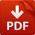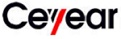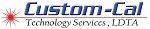PROGRAMMABLE STEP ATTENUATORS SELECTION GUIDE.

An attenuator is an electronic device that reduces the power of a signal without appreciably distorting its waveform.

An attenuator is effectively the opposite of an amplifier, though the two work by different methods. While an amplifier provides gain, an attenuator provides loss, or gain less than 1.

Attenuators are usually passive devices made from simple voltage divider networks. Switching between different resistances forms adjustable stepped attenuators and continuously adjustable ones using potentiometers. For higher frequencies precisely matched low VSWR resistance networks are used.

Fixed attenuators in circuits are used to lower voltage, dissipate power, and to improve impedance matching. In measuring signals, attenuator pads or adapters are used to lower the amplitude of the signal a known amount to enable measurements, or to protect the measuring device from signal levels that might damage it. Attenuators are also used to ‘match’ impedance by lowering apparent SWR (Standing Wave Ratio).

## Attenuator circuits

Basic circuits used in attenuators are pi pads (π-type) and T pads. These may be required to be balanced or unbalanced networks depending on whether the line geometry with which they are to be used is balanced or unbalanced. For instance, attenuators used with coaxial lines would be the unbalanced form while attenuators for use with twisted pair are required to be the balanced form.

Four fundamental attenuator circuit diagrams are given in the figures on the left. Since an attenuator circuit consists solely of passive resistor elements, it is both linear and reciprocal. If the circuit is also made symmetrical (this is usually the case since it is usually required that the input and output impedance Z1 and Z2 are equal), then the input and output ports are not distinguished, but by convention the left and right sides of the circuits are referred to as input and output, respectively.

Various tables and calculators are available that provide a means of determining the appropriate resistor values for achieving particular loss values. One of the earliest was published by the NAB in 1960 for losses ranging from 1/2 to 40 dB, for use in 600 ohm circuits.

## Attenuator characteristics

Key specifications for attenuators are:

• Attenuation expressed in decibels of relative power. A 3 dB pad reduces power to one half, 6 dB to one fourth, 10 dB to one tenth, 20 dB to one hundredth, 30 dB to one thousandth and so on. For voltage, you double the dBs so for example 6 dB is half in voltage.
• Nominal impedance, for example 50 ohm
• Frequency bandwidth, for example DC-18 GHz
• Power dissipation depends on mass and surface area of resistance material as well as possible additional cooling fins.
• SWR is the standing wave ratio for input and output ports
• Accuracy
• Repeatability

## RF attenuators

Radio frequency attenuators are typically coaxial in structure with precision connectors as ports and coaxial, micro strip or thin-film internal structure. Above SHF special waveguide structure is required.

Important characteristics are:

• accuracy,
• low SWR,
• flat frequency-response and
• repeatability.

The size and shape of the attenuator depends on its ability to dissipate power. RF attenuators are used as loads for and as known attenuation and protective dissipation of power in measuring RF signals.

## Audio attenuators

A line-level attenuator in the preamp or a power attenuator after the power amplifier uses electrical resistance to reduce the amplitude of the signal that reaches the speaker, reducing the volume of the output. A line-level attenuator has lower power handling, such as a 1/2-watt potentiometer or voltage divider and controls preamp level signals, whereas a power attenuator has higher power handling capability, such as 10 watts or more, and is used between the power amplifier and the speaker.

## Component values for resistive pads and attenuators

• All impedance, currents, voltages and two-port parameters will be assumed to be purely real. For practical applications, this assumption is often close enough.
• The pad is designed for a particular load impedance, ZLoad, and a particular source impedance, Zs.
• The impedance seen looking into the input port will be ZS if the output port is terminated by ZLoad.
• The impedance seen looking into the output port will be ZLoad if the input port is terminated by ZS.

### Reference figures for attenuator component calculationThis circuit is used for the general case, all T-pads, all pi-pads and L-pads when the source impedance is greater than or equal to the load impedance.The L-pad computation assumes that port 1 has the highest impedance. If the highest impedance happens to be the output port, then use this figure.

The attenuator two-port is generally bidirectional. However, in this section it will be treated as though it were one way. In general, either of the two figures applies, but the first figure (which depicts the source on the left) will be tacitly assumed most of the time. In the case of the L-pad, the second figure will be used if the load impedance is greater than the source impedance.

Each resistor in each type of pad discussed is given a unique designation to decrease confusion.

The L-pad component value calculation assumes that the design impedance for port 1 (on the left) is equal or higher than the design impedance for port 2.

### Terms used

• Input port will mean the input port of the two-port.
• Output port will mean the output port of the two-port.
• Symmetric means a case where the source and load have equal impedance.
• Loss means the ratio of power entering the input port of the pad divided by the power absorbed by the load.
• Insertion Loss means the ratio of power that would be delivered to the load if the load were directly connected to the source divided by the power absorbed by the load when connected through the pad.

### Symbols used

Passive, resistive pads and attenuators are bidirectional two-ports, but in this section they will be treated as unidirectional.

• ZS = the output impedance of the source.
• Zin = the impedance seen looking into the input port when ZLoad is connected to the output port. Zin is a function of the load impedance.
• Zout = the impedance seen looking into the output port when Zs is connected to the input port. Zout is a function of the source impedance.
• Vs = source open circuit or unloaded voltage.
• Vin = voltage applied to the input port by the source.
• Vout = voltage applied to the load by the output port.
• Iin = current entering the input port from the source.
• Iout = current entering the load from the output port.
• Pin = Vin Iin = power entering the input port from the source.
• Pout = Vout Iout = power absorbed by the load from the output port.
• Pdirect = the power that would be absorbed by the load if the load were connected directly to the source.
• Lpad = 10 log10 (Pin / Pout ) always. And if Zs = ZLoad then Lpad = 20 log10 (Vin / Vout ) also. Note, as defined, Loss ≥ 0 dB
• Linsertion = 10 log10 (Pdirect / Pout ). And if Zs = ZLoad then Linsertion = Lpad.

### Symmetric T pad resistor calculation

{\displaystyle A=10^{-Loss/20}\qquad R_{a}=R_{b}=Z_{S}{\frac {1-A}{1+A}}\qquad R_{c}={\frac {Z_{s}^{2}-R_{b}^{2}}{2R_{b}}}\qquad \,}see Valkenburg p 11-3

### Symmetric pi pad resistor calculation

{\displaystyle A=10^{-Loss/20}\qquad R_{x}=R_{y}=Z_{S}{\frac {1+A}{1-A}}\qquad R_{z}={\frac {2R_{x}}{\left({\frac {R_{x}}{Z_{S}}}\right)^{2}-1}}]\qquad \,}see Valkenburg p 11-3

### L-Pad for impedance matching resistor calculation

If a source and load are both resistive (i.e. Z1 and Z2 have zero or very small imaginary part) then a resistive L-pad can be used to match them to each other. As shown, either side of the L-pad can be the source or load, but the Z1 side must be the side with the higher impedance.

{\displaystyle R_{q}={\frac {Z_{m}}{\sqrt {\rho -1}}}\qquad R_{p}=Z_{m}{\sqrt {\rho -1}}}{\displaystyle {\text{Loss}}=20\log _{10}\left({\sqrt {\rho -1}}+{\sqrt {\rho }}\right)\quad {\text{where}}\quad \rho ={\frac {Z_{1}}{Z_{2}}}\quad Z_{m}={\sqrt {Z_{1}Z_{2}}}{\text{ }}\,}see Valkenburg p 11-3

Large positive numbers means loss is large. The loss is a monotonic function of the impedance ratio. Higher ratios require higher loss.

This is the Y-Δ transform

{\displaystyle R_{z}={\frac {R_{a}R_{b}+R_{a}R_{c}+R_{b}R_{c}}{R_{c}}}\qquad R_{x}={\frac {R_{a}R_{b}+R_{a}R_{c}+R_{b}R_{c}}{R_{b}}}\qquad R_{y}={\frac {R_{a}R_{b}+R_{a}R_{c}+R_{b}R_{c}}{R_{a}}}.\qquad \,}This is the Δ-Y transform

{\displaystyle R_{c}={\frac {R_{x}R_{y}}{R_{x}+R_{y}+R_{z}}}\qquad R_{a}={\frac {R_{z}R_{x}}{R_{x}+R_{y}+R_{z}}}\qquad R_{b}={\frac {R_{z}R_{y}}{R_{x}+R_{y}+R_{z}}}\qquad \,}### Conversion between two-ports and pads

The impedance parameters for a passive two-port are
{\displaystyle V_{1}=Z_{11}I_{1}+Z_{12}I_{2}\qquad V_{2}=Z_{21}I_{1}+Z_{22}I_{2}\qquad {\text{with}}\qquad Z_{12}=Z_{21}\,}It is always possible to represent a resistive t-pad as a two-port. The representation is particularly simple using impedance parameters as follows:
{\displaystyle Z_{21}=R_{c}\qquad Z_{11}=R_{c}+R_{a}\qquad Z_{22}=R_{c}+R_{b}\,}The preceding equations are trivially invertible, but if the loss is not enough, some of the t-pad components will have negative resistances.
{\displaystyle R_{c}=Z_{21}\qquad R_{a}=Z_{11}-Z_{21}\qquad R_{b}=Z_{22}-Z_{21}\,}{\displaystyle R_{z}={\frac {Z_{11}Z_{22}-Z_{21}^{2}}{Z_{21}}}\qquad R_{x}={\frac {Z_{11}Z_{22}-Z_{21}^{2}}{Z_{22}-Z_{21}}}\qquad R_{y}={\frac {Z_{11}Z_{22}-Z_{21}^{2}}{Z_{11}-Z_{21}}}\qquad }The admittance parameters for a passive two port are
{\displaystyle I_{1}=Y_{11}V_{1}+Y_{12}V_{2}\qquad I_{2}=Y_{21}V_{1}+Y_{22}V_{2}\qquad {\text{with}}\qquad Y_{12}=Y_{21}\,}It is always possible to represent a resistive pi pad as a two-port. The representation is particularly simple using admittance parameters as follows:
{\displaystyle Y_{21}={\frac {1}{R_{z}}}\qquad Y_{11}={\frac {1}{R_{x}}}+{\frac {1}{R_{z}}}\qquad Y_{22}={\frac {1}{R_{y}}}+{\frac {1}{R_{z}}}\,}The preceding equations are trivially invertible, but if the loss is not enough, some of the pi-pad components will have negative resistances.
{\displaystyle R_{z}={\frac {1}{Y_{21}}}\qquad R_{x}={\frac {1}{Y_{11}-Y_{21}}}\qquad R_{y}={\frac {1}{Y_{22}-Y_{21}}}\,}### General case, determining impedance parameters from requirements

Because the pad is entirely made from resistors, it must have a certain minimum loss to match source and load if they are not equal.

The minimum loss is given by

{\displaystyle Loss_{min}=20\ log_{10}\left({\sqrt {\rho -1}}+{\sqrt {\rho }}\quad \right)\,\quad {\text{where}}\quad \rho ={\frac {\max[Z_{S},Z_{Load}]}{\min[Z_{S},Z_{Load}]}}\,}Although a passive matching two-port can have less loss, if it does it will not be convertible to a resistive attenuator pad.

{\displaystyle A=10^{-Loss/20}\qquad Z_{11}=Z_{S}{\frac {1+A^{2}}{1-A^{2}}}\qquad Z_{22}=Z_{Load}{\frac {1+A^{2}}{1-A^{2}}}\qquad Z_{21}=2{\frac {A{\sqrt {Z_{S}Z_{Load}}}}{1-A^{2}}}\,}Once these parameters have been determined, they can be implemented as a T or pi pad as discussed above

• ### 81501 Programmable Step Attenuator DC 18GHz

• Attenuation can be set up manually through front panel, or be controlled through rear panel GPIB
• Maximum attenuation is up to 121 dB
• Attenuation steeping is adjustable, minimum is 1 dB
• Front panel shows the real-time attenuation; convenient for useProgrammable Attennuator

$3,750.00 Brands:• ### 81504 Programmable step attenuator / DC-40 GHz / 0-115 db • Frequency up to 67 GHz, maximum input power up to 50 W • Broad bandwidth, low SWR, high attenuation accuracy • Maximum attenuation up to 115 dB, minimum step size is 1 dBStep Attenuator$1,470.00
Brands:• ### 81505 Programmable step attenuator / DC-50 GHz / 0-10 dB

• Frequency up to 67 GHz, maximum input power up to 50 W
• Broad bandwidth, low SWR, high attenuation accuracy
• Maximum attenuation up to 115 dB, minimum step size is 1 dBStep Attenuator
Call for Price
Brands:• ### 81506 Programmable step attenuator / DC-40 GHz / 0-90 db

• Frequency up to 67 GHz, maximum input power up to 50 W
• Broad bandwidth, low SWR, high attenuation accuracy
• Maximum attenuation up to 115 dB, minimum step size is 1 dBStep Attenuator
Call for Price
Brands:• ### 81507 Programmable step attenuator / DC-40 GHz / 0-60 db

• Frequency up to 67 GHz, maximum input power up to 50 W
• Broad bandwidth, low SWR, high attenuation accuracy
• Maximum attenuation up to 115 dB, minimum step size is 1 dBStep Attenuator
Call for Price
Brands:• ### 81508 Programmable step attenuator / DC-50 GHz / 0-60 dB

• Frequency up to 67 GHz, maximum input power up to 50 W
• Broad bandwidth, low SWR, high attenuation accuracy
• Maximum attenuation up to 115 dB, minimum step size is 1 dBStep Attenuator
Call for Price
Brands:• ### 81510 Programmable step attenuator / DC-26,5 GHz / 0-11 dB

• Frequency up to 67 GHz, maximum input power up to 50 W
• Broad bandwidth, low SWR, high attenuation accuracy
• Maximum attenuation up to 115 dB, minimum step size is 1 dBStep Attenuator
Call for Price
Brands:• ### 81510F Programmable step attenuator / DC-40 GHz / 0-11 db

• Frequency up to 67 GHz, maximum input power up to 50 W
• Broad bandwidth, low SWR, high attenuation accuracy
• Maximum attenuation up to 115 dB, minimum step size is 1 dBStep Attenuator
Call for Price
Brands:• ### 81511 Programmable step attenuator / DC-40 GHz / 0-70 dB

• Frequency up to 67 GHz, maximum input power up to 50 W
• Broad bandwidth, low SWR, high attenuation accuracy
• Maximum attenuation up to 115 dB, minimum step size is 1 dBStep Attenuator
\$1,376.00
Brands:• ### 81512 Programmable step attenuator / DC-40 GHz / 0-35 dB

• Frequency up to 67 GHz, maximum input power up to 50 W
• Broad bandwidth, low SWR, high attenuation accuracy
• Maximum attenuation up to 115 dB, minimum step size is 1 dBStep Attenuator
Call for Price
Brands:• ### 81512A Programmable step attenuator / DC-50 GHz / 0-35 dB

• Frequency up to 67 GHz, maximum input power up to 50 W
• Broad bandwidth, low SWR, high attenuation accuracy
• Maximum attenuation up to 115 dB, minimum step size is 1 dBStep Attenuator
Call for Price
Brands:• ### 81514 Programmable step attenuator / DC-40 GHz / 0-75 dB

• Frequency up to 67 GHz, maximum input power up to 50 W
• Broad bandwidth, low SWR, high attenuation accuracy
• Maximum attenuation up to 115 dB, minimum step size is 1 dBStep Attenuator
Call for Price
Brands:• ### 81515 Programmable step attenuator / DC-40 GHz / 0-110 dB

• Frequency up to 67 GHz, maximum input power up to 50 W
• Broad bandwidth, low SWR, high attenuation accuracy
• Maximum attenuation up to 115 dB, minimum step size is 1 dBStep Attenuator
Call for Price
Brands:• ### 81516 Programmable step attenuator / DC-26,5 GHz / 0-70 dB

• Frequency up to 67 GHz, maximum input power up to 50 W
• Broad bandwidth, low SWR, high attenuation accuracy
• Maximum attenuation up to 115 dB, minimum step size is 1 dBStep Attenuator
Call for Price
Brands:• ### 81517 Programmable step attenuator / DC-26,5 GHz / 0-75 dB

• Frequency up to 67 GHz, maximum input power up to 50 W
• Broad bandwidth, low SWR, high attenuation accuracy
• Maximum attenuation up to 115 dB, minimum step size is 1 dBStep Attenuator
Call for Price
• ### 81518 Programmable step attenuator / DC-26,5 GHz / 0-110 dB

• Frequency up to 67 GHz, maximum input power up to 50 W
• Broad bandwidth, low SWR, high attenuation accuracy
• Maximum attenuation up to 115 dB, minimum step size is 1 dBStep Attenuator
Call for Price
Brands:• ### 81519 Programmable step attenuator / DC-26,5 GHz / 0-35 dB

• Frequency up to 67 GHz, maximum input power up to 50 W
• Broad bandwidth, low SWR, high attenuation accuracy
• Maximum attenuation up to 115 dB, minimum step size is 1 dBStep Attenuator
Call for Price
Brands:• ### 81524D Programmable Step Attenuator DC 18GHz

• Attenuation can be set up manually through front panel, or be controlled through rear panel GPIB
• Maximum attenuation is up to 121 dB
• Attenuation steeping is adjustable, minimum is 1 dB
• Front panel shows the real-time attenuation; convenient for useProgrammable Attennuator

Call for Price
Brands:• ### 81525D Programmable Step Attenuator DC 18GHz

• Attenuation can be set up manually through front panel, or be controlled through rear panel GPIB
• Maximum attenuation is up to 121 dB
• Attenuation steeping is adjustable, minimum is 1 dB
• Front panel shows the real-time attenuation; convenient for useProgrammable Attennuator

Call for Price
Brands: